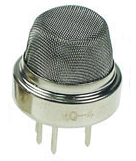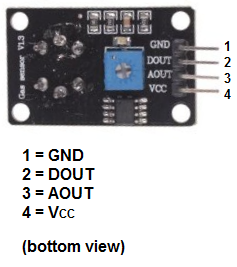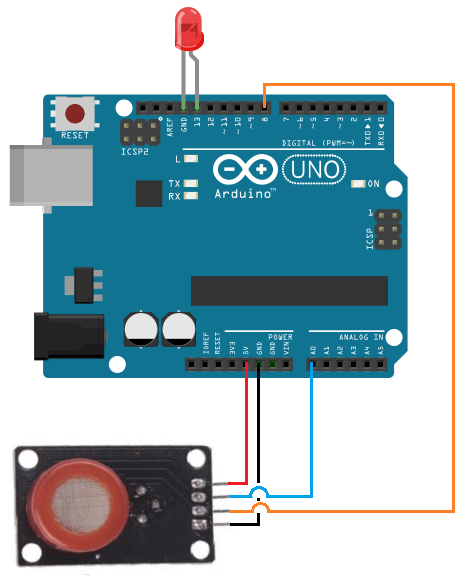﻿ MQ-4 Methane Sensor Circuit Built with an Arduino ﻿# MQ-4 Methane Sensor Circuit Built with an ArduinoIn this project, we will go over how to build a methane sensor circuit with an arduino.

The methane sensor we will use is the MQ-4 sensor. This is a sensor that is sensitive to effects of methane.

Methane (CH4) is a colorless, odorless gas with a wide distribution in nature. Methane is known chiefly as being the principal component of natural gas, which is a mixture containing about 75% methane (CH4), 15% ethane (C2H6), and 5% other hydrocarbons, suh as propane (C3H8) and butane (CH4H10).

The principal use of methane is as a fuel. The combustion of methane is highly exothermic, meaning reactions create the release of a great deal of energy. The energy released by the combustion of methane, in the form of natural gas, is used directly to heat homes and commercial buildings. It is also used in the generation of electric power. During the past decade, natural gas accounted for about 1/5 of the total energy consumption worldwide and about 1/3 in the United States.

Much debate has been stirred on whether natural gas is more green, meaning better for the environment, than coal. This is because methane, when burned, emits less carbon dioxide than coal. Carbon dioxide, CO2, is a dangerous greenhouse gas. However, when unburned, when it is vented or leaked directly into the atmosphere, methane is far more potent than carbon dioxide. In terms of its contribution to global warming, it is 23 times more powerful than carbon dioxide.

Thus, methane is a powerful gas that can do a lot of good and potentially a lot of bad at the same time.

Therefore, it's a gas that's important to monitor.

And to do so, we need a methane sensor. So this is the device we will build in this project. OUr device will be able to detect the gas methane and tell us its concentration in the air.

### Components Needed

• MQ-4 Methane Sensor
• Arduino
• LED

The MQ-4 can be obtained very cheaply, just a few bucks. A good place to look for it is on ebay, which always has auctions on them for the \$2-\$3 range.

Important, it is recommended that you do not obtain the standalone sensor but the whole MQ-4 board. This is because if you buy the standalone sensor, you will have to finish building the whole schematic before you can connect it to the arduino. So that less work is required for integrating it with the arduino, it is recommended that you buy the complete MQ-4 sensor circuit. This you can see below.

If you buy the complete board, there are 4 leads which need to be connected.There 4 leads are +5V, AOUT, DOUT, and GND.

The +5V and GND leads establishes power for the alcohol sensor.

The other 2 leads are AOUT (analog output) and DOUT (digital output). How the sensor works is the terminal AOUT gives an analog voltage output in proportion to the amount of methane the sensor detects. The more methane it detects, the greater the analog voltage it will output. Conversely, the less CO it detects, the less analog voltage it will output. If the analog voltage reaches a certain threshold, it will send the digital pin DOUT high. Once this DOUT pin goes high, the arduino will detect this and will trigger the LED to turn on, signaling that the methane threshold has been reached and is now over the limit. How you can change this threshold level is by adjusting the potentiometer to either raise or lower the level.

### MQ-4 Methane Sensor Circuit Schematic

The methane sensor circuit we will build with an MQ-4 sensor integrated with an arduino is shown below.The connections are pretty basic.

To connect the sensor, there are 4 leads. 2 of them are for power. The +5V terminal of the sensor connects into the 5V terminal of the arduino board. The GND terminal of the sensor connects into the GND terminal of the arduino. This establishes power for the sensor.

The other 2 connections are the analog and digital output of the sensor. These connect to analog pin A0 and digital pin D8, respectively.

### Code

The code which we need to upload to the arduino so that it can measure methane levels is shown below.

/* MQ-4 Methane Sensor Circuit with Arduino */

const int AOUTpin=0;//the AOUT pin of the methane sensor goes into analog pin A0 of the arduino
const int DOUTpin=8;//the DOUT pin of the methane sensor goes into digital pin D8 of the arduino
const int ledPin=13;//the anode of the LED connects to digital pin D13 of the arduino

int limit;
int value;

void setup() {
Serial.begin(115200);//sets the baud rate
pinMode(DOUTpin, INPUT);//sets the pin as an input to the arduino
pinMode(ledPin, OUTPUT);//sets the pin as an output of the arduino
}

void loop()
{
Serial.print("Methane value: ");
Serial.println(value);//prints the methane value
Serial.print("Limit: ");
Serial.print(limit);//prints the limit reached as either LOW or HIGH (above or underneath)
delay(100);
if (limit == HIGH){
digitalWrite(ledPin, HIGH);//if limit has been reached, LED turns on as status indicator
}
else{
digitalWrite(ledPin, LOW);//if threshold not reached, LED remains off
}
}

The first block of code defines all the pin connections of the sensor and the LED. Since the AOUTpin connects to analog pin A0, it is initialized to 0. Since the DOUTpin connects to digital pin D8, it is initialized to 8. Since the LED connects to digital pin D13, it is initialized to 13. 2 variables, limit and value, are also declared. These will be used to store the value of the analog pin AOUT and digital pin DOUT.

The next block of code sets the baud rate and declares the DOUTpin as input and the ledPin as output. This is because the sensor is an input to the arduino for the arduino to read and process the sensor value. And the LED is an output will serves an indicator if the sensor has detected methane.

The next block of code reads the sensor pin AOUT and stores the value in the integer value. It also reads the sensor pin DOUT and stores the value in the integer limit. We then print the alcohol value, which will be a numeric value ranging from either 0 (no alcohol detected) to 1023 (maximum level of methane that can be read). We will aslo print the limit which will either be HIGH or LOW. If the methane detected is under the threshold level, the value of limit returned will be low. If the methane detected is above the threshold, the value of limit returned will be HIGH.

If the value is HIGH, the LED will turn on. If the value is low, the LED will remain off.

Related Resources